# 通过预定义 Schema 提高 eKuiper 数据处理吞吐eKuiper Team
2023-8-31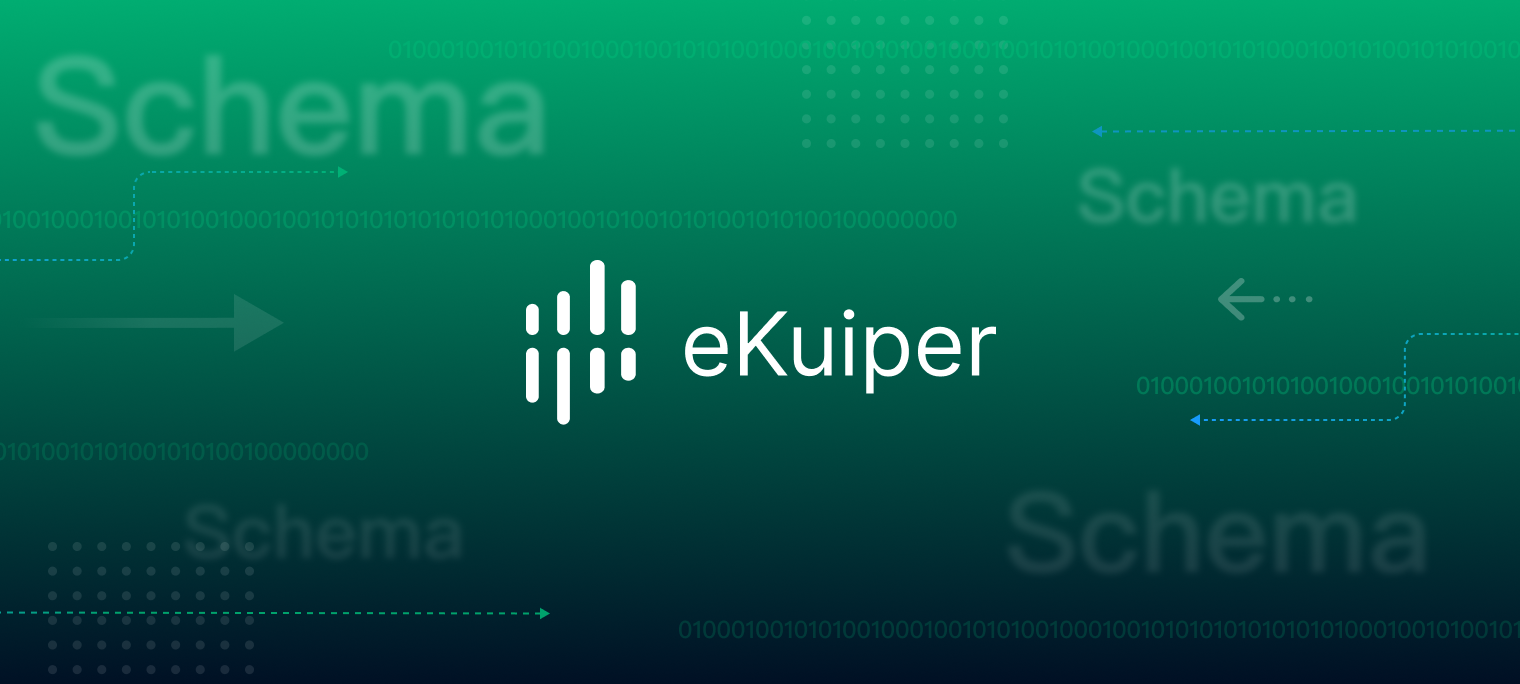## 通过 Schema 预检验数据

``````my_stream (id bigint, name string, score float)WITH ( datasource = "topic/temperature", FORMAT = "json", KEY = "id");
``````

``````select id, name from my_stream where score > 60
``````

``````select id, name from my_stream where email = 'mock'
``````

``````unknown field email
``````

``````{
"id": 1,
"name": 12,
"score": 75
}
``````

## 解析 Schemaless 数据

``````func (c *Converter) Decode(b []byte) (interface{}, error) {
var r0 interface{}
err := json.Unmarshal(b, &r0)
if err != nil {
return nil, err
}
return r0, nil
}
``````

``````select a1,a2,a3,a4,a5 from my_stream;
``````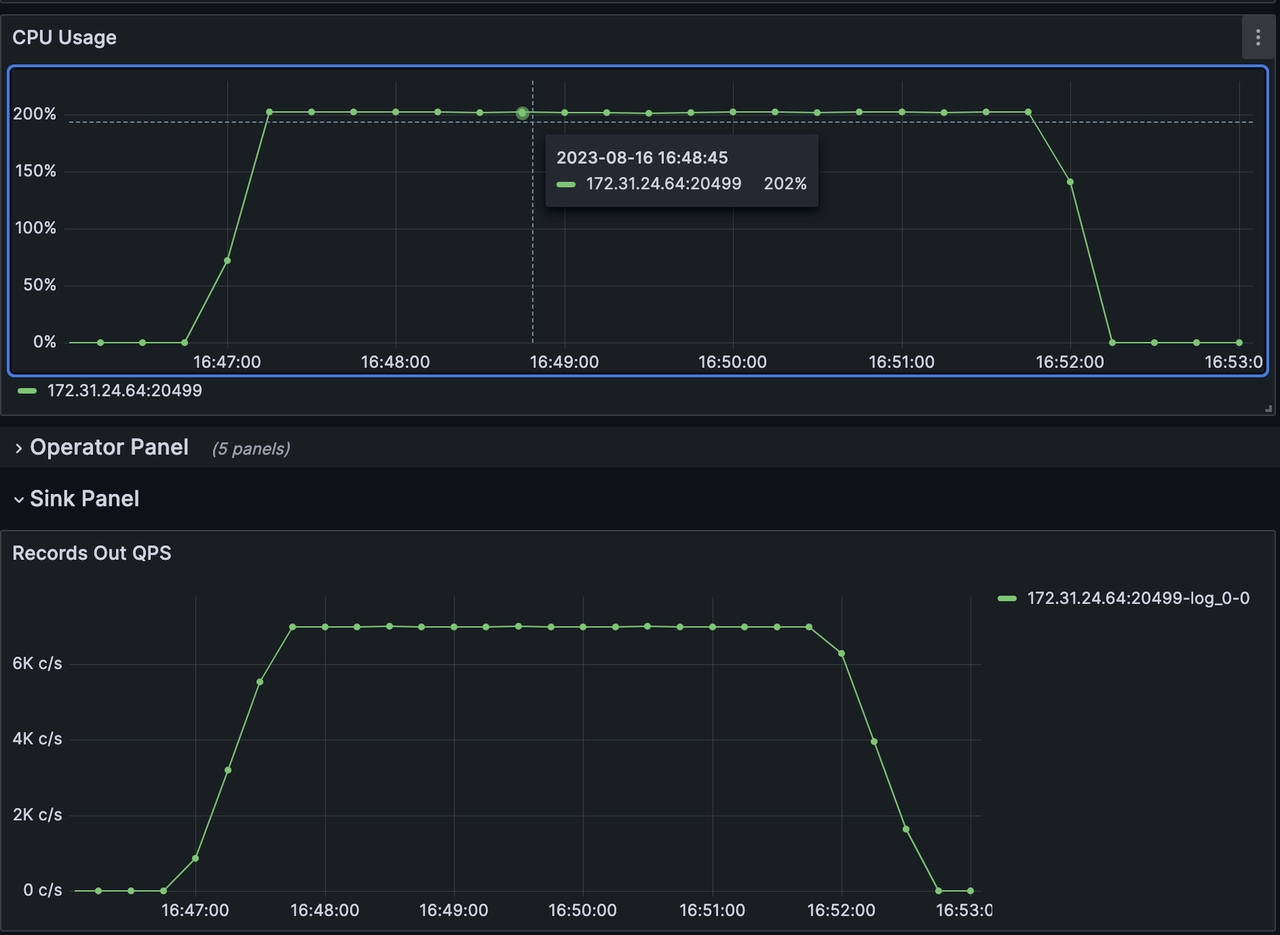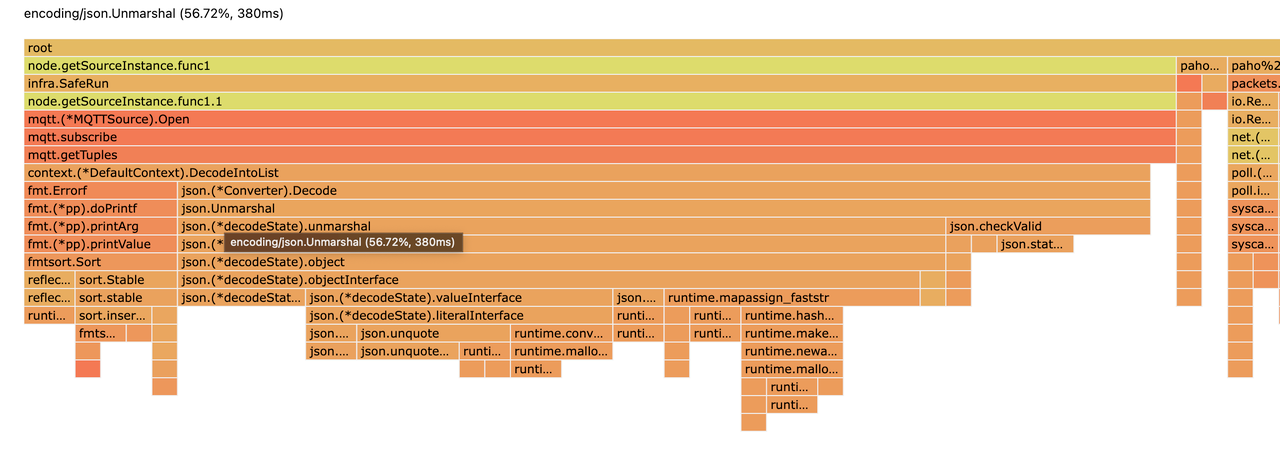### 预定义 Schema

``````create stream my_stream '(a1 string, a2 string, a3 string) WITH (FORMAT="JSON", DATASOURCE="test")'
``````
``````{
"a1": "sdafasdf",
"a2": "zxczxczx",
"a3": "weqeqwee",
"a4": "sdfsdfsf",
}
``````

### 列裁剪

``````select a1,a2 from my_stream
``````
``````select a2,a3 from my_stream
``````

### fastjson

``````        var p fastjson.Parserv, err := p.Parse(`{
"str": "bar",
"int": 123,
"float": 1.23,
"bool": true,
"arr": [1, "foo", {}]
}`)
if err != nil {
log.Fatal(err)
}
fmt.Printf("foo=%s\n", v.GetStringBytes("str"))
fmt.Printf("int=%d\n", v.GetInt("int"))
fmt.Printf("float=%f\n", v.GetFloat64("float"))
fmt.Printf("bool=%v\n", v.GetBool("bool"))
fmt.Printf("arr.1=%s\n", v.GetStringBytes("arr", "1"))
``````

### 优化结果

``````create stream my_stream '(a1 string, a2 string,a3 string,a4 string, a5 string) WITH (FORMAT="JSON", DATASOURCE="test")'
``````

``````select a1,a2,a3,a4,a5 from my_stream;
``````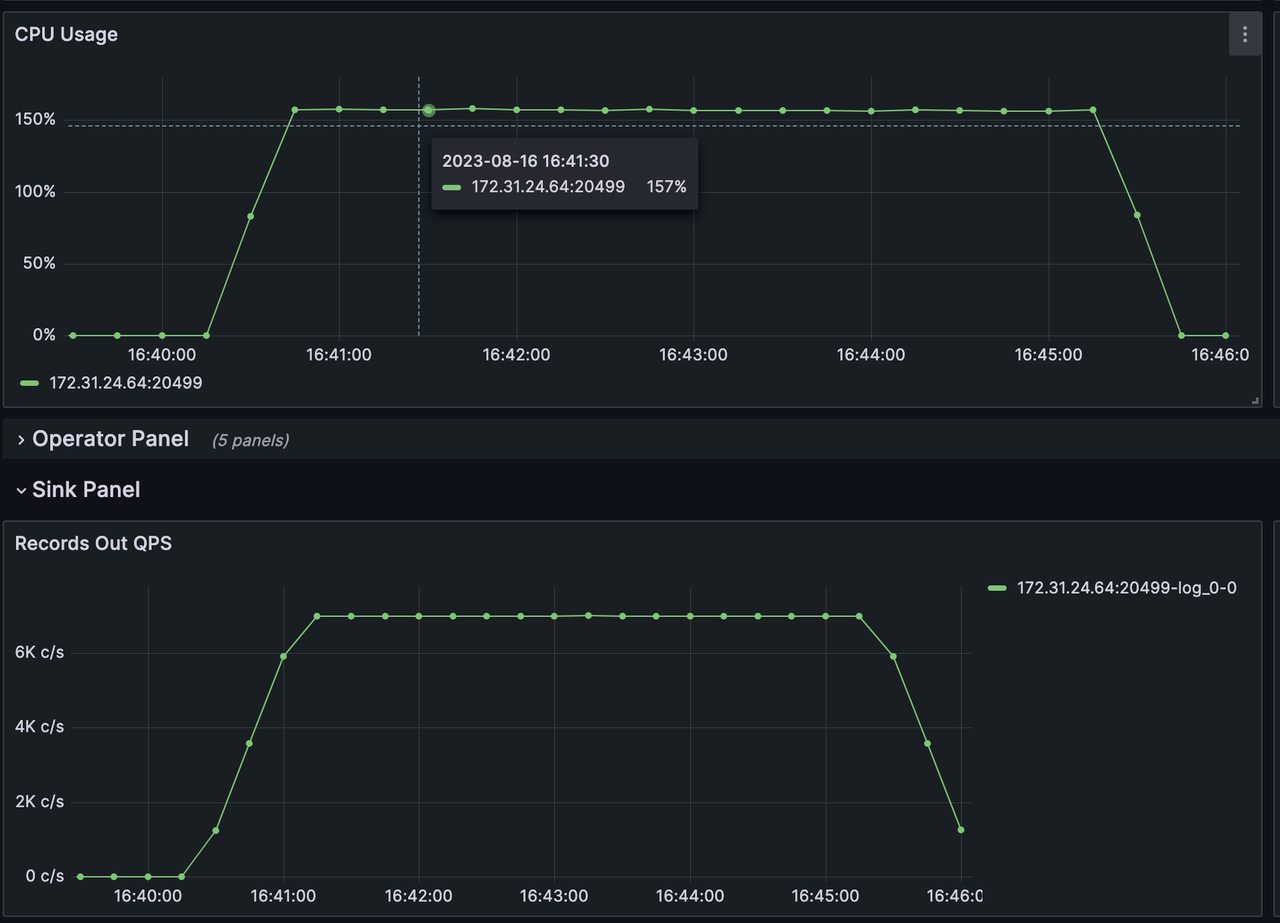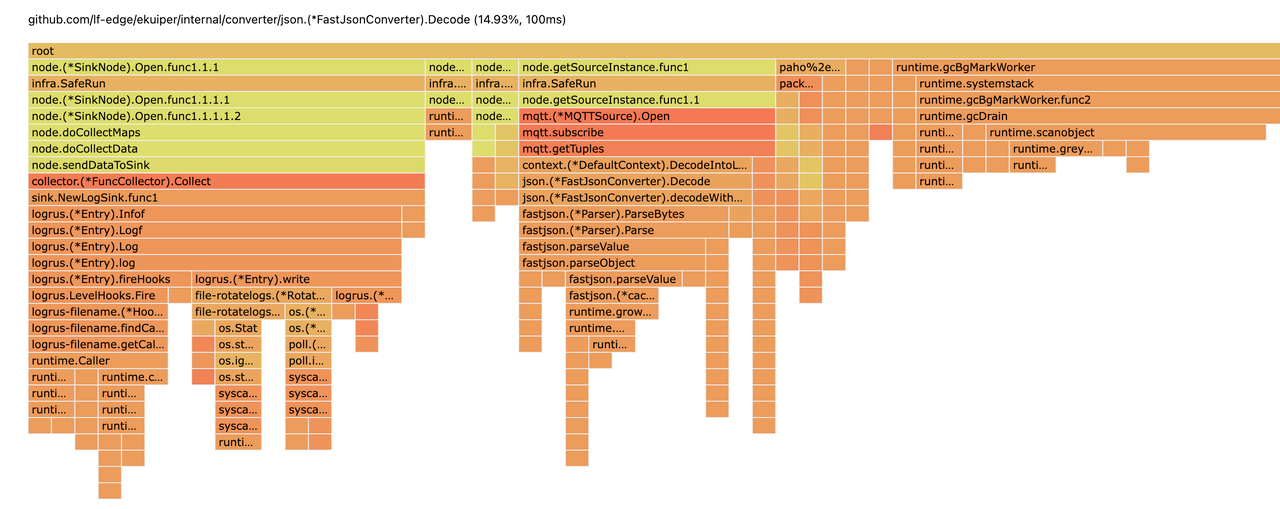## 推荐阅读

2019-11-27eKuiper Team